Short Questions and Answers on Amplitude Modulation

Q.1. Write the expression for AM wave?

Ans. The standard equation for amplitude modulated (AM) wave may be expressed as :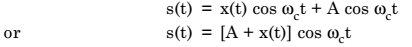Q.2. What is the envelope of AM wave?

Ans. The expression for AM wave is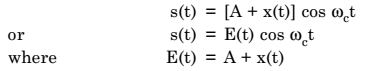E(t) is called the envelope of AM wave. This envelope consists of the baseband signal x(t). Hence, the modulating or baseband signal may be recovered from an AM wave by detecting the envelope.

Q.3. What is the BW for AM wave?

Ans. The difference between these two extreme frequencies is equal to the bandwidth of the AM wave. Therefore,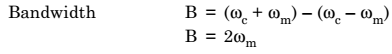Q.4. What are the frequency components in an AM wave?

Ans. The AM signal has three frequency components as follow: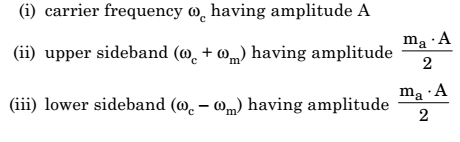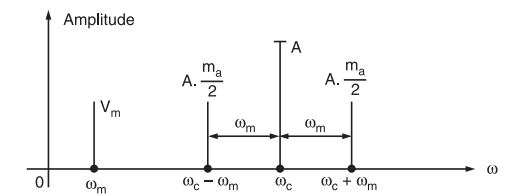Fig. 1: Single-sided frequency spectrum of single-tone AM wave

With the help of these frequency components, we can plot the frequency-spectrum of single-tone amplittude modulated (AM) wave. Figure 1 shows the one-sided frequency spectrum of single-tone AM wave.

Q.5. Define transmission efficiency in AM wave?

Ans. In AM wave, the amount of useful message power Ps may be expressed by a term known as transmission efficiency h.

Q.6. What is demodulation?

Ans. The process of extracting a modulating or baseband signal from the modulated signal is called demodulation or detection.

In other words, demodulation or detection is the process by which the message is recovered from the modulated signal at receiver.

The devices used for demodulation or detection are called demodulators or detectors.

For amplitude modulation, detectors or demodulators are categorized as: (i) Square-Law detectors, (ii) Envelope detectors.

Q.7. How can you obtain a DSB-SC signal?

Ans. A DSB-SC signal is obtained by simply multiplying modulating signal x(t) with carrier signal cos ωct. This is achieved by a product modulator. The block diagram of a product modulator is shown in figure 2.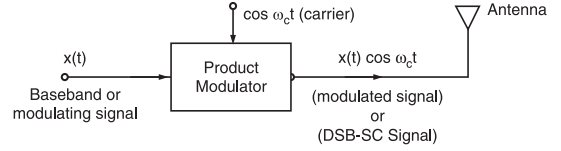Fig. 2:

Q.8. What is the BW of DSB-SC signal?

Ans. Bandwidth,

B = (ωc + ωm) – (ωc – ωm) = 2 ωm

It is obvious that the bandwidth of DSB-SC modulation is same as that of general AM wave.

Q.9. What are the demodulation methods for DSB-SC signal?

Ans. The DSB-SC signal may be demodulated by following two methods:

(i) Synchronous detection method

(ii) Using envelope detector after carrier re-insertion.

Q.10. What are the generating methods for SSB-SC signal.

Ans. SSB-SC signals may be generated by two methods as under:

(i) Frequency discrimination method or filter method

(ii) Phase discrimination method or phase-shift method

Q.11. What do you mean by multiplexing?

Ans. Multiplexing is a technique in which several message signals are combined into a composite signal for transmission over a common channel.

In order to transmit a number of these signals over the same channel, the signals must be kept apart so that they do not interfere with each other, and hence they can be separated easily at the receiver end.

Q.12. What all frequency components are present in AM wave spectrum?

Ans. Carrier fc, upper sideband (fc+fm) and lower sideband (fc –fm).

Q.13. What is the maximum value of m for distortion free transmission?

Ans. Ideally maximum value of m is 1.

Q.14. Why do we consider the rms carrier voltage to calculate the carrier power?

Ans. Because rms value decides the amount of power dissipated in the radiation resistance R of an antenna.

Q.15. How is the information transmitted using AM?

Ans. By varying the instantaneous amplitude of the carrier in proportion with the instantaneous amplitude of the message signal or information.

Q.16. What is the other name of AM?

Ans. DSB-FC i.e., double sideband ful carrier.

Q.17. Why is an AM signal get severely affected due to noise?

Ans. Because the information is contained in the amplitude variations of an AM wave, and the noise modifies the amplitude of the AM signal. Thus due to noise the information will be contaminated.

Q.18. What is the difference between percent modulation and modulation index?

Ans. Percent modulation is modulation index expressed as percentage.

Q.19. Name different types of filters used for filter method.

Ans. LC filters, crystal filters, ceramic filters, mechanical filters. The mechanical filter has the best properties such as small size, good passband and good attenuation characteristics.

Q.20. Write down the expression for an AM wave.

Q.21. Write down the expression for carrier power.

Ans.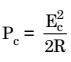Q.22. What is the minimum bandwidth of an AM wave?

Ans. 2 fm

Q.23. Write the expression for sideband power in AM.

Q.24. How do we vary the amplitude of sidebands?

Ans. By varying the modulation index m, if carrier amplitude is constant because amplitude of each sideband =

m Ec/2.

Q.25. Define modulation index of an AM wave.

Ans. It is the ratio of peak amplitudes of modulating signal and carrier i.e.,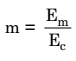Q.26. When does overmodulation take place and what is its effect?

Ans. Overmodulation takes place when m > 1. A part of the AM wave which crosses the zero axis (X axis) will disappear due to overmodulation.

Q.27. What is the maximum value of sideband power in AM?

Ans. Maximum power in each sideband = Pc / 4 (at m = 1).

Q.28. What is the maximum transmitted power in AM?

Ans. The maximum transmitted power Pt(max) = 1.5 Pc (at m = 1).

Q.29. Define transmission efficiency. What is its maximum value for AM?

Ans. It is the ratio of transmitted power which contains information to the total transmitted power.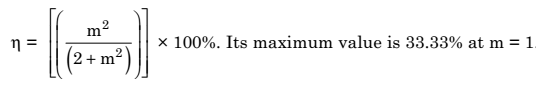Q.30. Write the expression for total modulation index for simultaneous modulation by “n” modulating signals.

Q.31. What is the effect of m on the carrier power in AM?

Ans. No effect, Pc remains constant independent of m.

Q.32. Where is the AM used?

Ans. AM is exclusively used for radio broadcasting applications. For example, Pune A station (Aakashwani Pune) uses AM for broadcasting its programs.

Q.33. For a trapezoidal pattern displayed on the CRO screen, what is the expression for the modulation index?

Q.34. What is the bandwidth of SSB signal?

Ans. fm Hz.

Q.35. Express the total sideband power as a percentage of the carrier power for 100% modulation.

Ans.

1. Simplicity
2. cost efficient
3. AM can travel long distance
4. smaller BW as compared to FM.

Q.37. Which of these frequency components contain the message information?

Ans. Only the upper and lower sidebands.

Q.38. State the application areas of SSB.

Ans. Land and air mobile communication, telemetry, military applications, navigation and a amateur radio are the application areas of SSB.

Q.39. Why should be carrier be a pure sine wave and should not contain any harmonics?

Ans. Because the harmonics of the carrier will be treated as “stations” by a receiver. So they will interfere with the already existing stations at or near these frequencies.

Q.40. State applications of AM.

Q.41. For AM with multiple sine wave modulating signals the number of sidebands is equal to……..

Ans. Twice the number of modulating signals.

Q.42. Why is SSB preferred over DSBFC or DSBSC?

Ans. Due to power saving, reduced channel bandwidth and reduced interference of noise, SSB is preferred.

Q.43. State the principle of balanced modulator.

Ans. If two signals at different frequencies are passed through a nonlinear resistance then at the output, we get an AM signal with suppressed carrier (DBSC).

Q.44. Name the devices which possess nonlinear resistance.

Ans. Diodes, BJT, JFET.

Q.45. For an AM signal represented by eAM = 10 (1 + 0.4 cos 6280 t) cos (6.28 × 106 t). What are the values of modulation index and carrier frequency?

Ans. m = 0.4, fc = 1 × 106Hz = 1 MHz.

Q.46. How to differentiate between DSB and AM?

Ans. DSB signal has a 180° phase shift at the zero crossing points. AM does not show any such phase shift.

Q.47. For a carrier signal of 1 MHz and modulating signal of 2 kHz what is the frequency range occupied by the AM signal?

Ans. 998 kHz to 1002 kHz.

Q.48. If f1 and f2 are the input frequencies to an ideal balanced modulator, what are the frequencies at its output?

Ans. If f1 > f2, then, output frequencies will be (f1 + f2) and (f1 – f2), provided that f2 is filtered out.

Q.49. Name two different methods used for removing the unwanted sideband.

Ans. Filter method, phase shift method.

Q.50. The AM signal without carrier is called as………..

Ans. DSBSC signal.

Q.51. A typical application of DSB is……..

Ans. Two way communication.

Q.52. Why is SSB not used for broadcasting?

Ans. SSB is not used for broadcasting due to following reasons:

1. As SSB transmitter and receivers are required to have excellent frequency stability.

2. It is not possible to design a tunable local oscillator with very high frequency stability.

Q.53. Why is the modulation carried out at low frequency in filter method?

Ans. For successful attenuation of the unwanted sideband, it is necessary to have a sharp filter response. Practically, it is easier to obtain such a response at low operating frequencies. Hence, the modulation is carried out at low frequency.

Q.54. What do you understand by the term pilot carrier?

Ans. A weakened carrier sent along with SSB signal is called as pilot carrier.

Q.55. What is the difference between SSB and ISB?

Ans. In ISB (independent sideband system), the sidebands generated about the pilot carrier are completely independent from each other and they can simultaneously convey totally different information.

Q.56. Is the carrier fully suppressed in ISB?

Ans. No, It is partially suppressed.

Q.57. What is the bandwidth of ISB system?

Ans. Bandwidth = ( fm1 + fm2 )

Q.58. For an ISB system with 6 kHz bandwidth per channel, how many voice channels can be accommodated per sideband?

Ans. Two

Q.59. What is the advantage of using VSB over SSB?

Ans. Due to partial transmission of the unwanted sideband in VSB, the design of practical filter for elimination of unwanted sideband becomes easier. It is not so in SSB.

Q.60. What is the function of the pilot carrier?

Ans. The pilot carrier acts as a reference signal to help the demodulation process in the receiver.

Q.61. What are the advantages of using VSB?

Ans. (i) Reduced bandwidth as compared to DSBFC system.

(ii) Practical filters can be used.

Q.62. How do you differentiate between DSBFC and DSBSC signals displayed on the CRO?

Ans. In DSBSC signal, we observe a phase reversal. Such a phase reversal is not seen for the DSBFC signal.

Q.63. State applications of VSB transmission.

Ans. VSB is most popularly used in the TV transmission.

Q.64. VSB is AM, FM or some other type of modulation?

Ans. VSB is basically AM.

Q.65. Can we use a simple resistive network as balanced modulator?

Ans. No, Because the transfer characteristics of a resistive network will be linear.

Q.66. In the phase shift method, how is the upper sideband suppressed?

Ans. By applying the modulating and carrier signals to the upper balanced modulator directly (without any

Q.67. Why are linear amplifiers used in SSB and ISB transmitters?

Ans. Linear amplifiers are used to amplify a signal without distorting its shape.

Q.68. Name a nonlinear amplifier.

Ans. Class C amplifier is a non-linear amplifier.

Q.69. Why is the amplitude of sound carrier less than that of a picture carrier in TV transmission?

Ans. If the amplitude of sound carrier is comparable with that of the picture carrier, then it will create a visible disturbance on the TV screen. This happens due to the interference of strong sound carrier. To avoid this, the amplitude of the sound carrier is reduced to about 10% of the picture carrier amplitude.

Q.70. In the diode ring modulator, the diode act as…………..

Ans. Switches

Q.71. What are the technical names of SSBRC (SSB with reduced carrier) and SSBSC (SSB with suppressed carrier)?

Ans. The technical names are: SSBRC: R3E and SSBSC: J3E.

Q.72. The output of an ideal balanced modulator is……..

Ans. DSB signal.

Q.73. The inputs to a balanced modulator are 1 MHz and a carrier of 2 MHz. The outputs are……..

Ans. 1 MHz and 3 MHz i.e. LSB and USB.

Q.74. Frequency translation is done with a circuit called as………

Ans. Mixer.

Q.75. During modulation, the information signal is called as ………. and the high frequency signal is called as…

Ans. Modulating signal and carrier.

Q.76. The carrier frequency is usually ………. than the modulating frequency.

Ans. Higher

Q.77. Express the total sideband power as a percentage of the carrier power for 100% modulation.

Q.78. The outline of the peaks of the carrier signal is called …………. and it has the same shape as the …………..

Ans. Envelope, modulating signal.

Q.79. The carrier frequency in AM is….

Ans. Constant

Q.80. The depth of modulation is given by

Ans. m × 100%

Q.81. How is plate modulator superior to the grid modulator?

Ans. Because, it does not produce any distortion, it has higher efficiency and higher output power as compared to the grid modulator.

Q.82. Why is grid leak bias is used in plate modulated class C amplifier?

Ans. For controlling the grid current.

Q.83. Why is an AM signal get severely affected due to noise?

Ans. Because the information is contained in the amplitude variations of an AM wave, and the noise modifies the amplitude of the AM signal. Thus, due to noise, the information will be contaminated.

Q.84. To achieve 50 percent modulation with carrier ec= 20 cos ωct, the modulating signal amplitude Em should be……..

Ans. 10 V

Q.85. The ideal value of m for maximum amplitude of modulating signal is……..

Ans. 1

Q.86. A signal whose amplitude is displayed with respect to time is called as…….

Ans. Time domain display

Q.87. A signal whose amplitude is displayed with respect to frequency is called as….

Ans. Frequency domain display

Q.88. The total transmitted power in AM is the sum of …….. and ………..

Ans. Carrier power and total side band power.

Q.89. An AM signal with a carrier of 1 kw has 100w power in each side band. The modulation index is…..

Ans. 0.6324

Q.90. Expression for AM with nonsinusoidal modulating signal is given by…

Q.91. What type of modulation is used in TV transmission?

Ans. Low level IF modulation.

Ans.

1. Larger BW requirement
2. Large power requirement

Q.93. What is the difference between high and low level modulation?

Ans. In low level modulation, the modulation takes place at a low power level. The power level of modulated signal is then raised to the adequate value, using amplifiers. In high level modulation, the signals are amplified first and then modulation takes place at a high power level.

Q.94. What type of amplifier is used for collector modulator?

Ans. Class C amplifier.

Q.95. Where is the operating point of class C amplifier located?

Ans. Below cut off.

Q.96. Name different linear amplifiers.

Ans. Class A, B and AB are linear amplifiers.

Q.97. In collector modulation, how do you obtain the AM wave across the tank circuit from unidirectional collector current pulses?

Ans. When current pulses are supplied to the tank circuit, it produces damped voltage oscillations. The amplitude of these voltage oscillations is proportional to the amplitude of current pulses supplied. This principle is used for producing AM in collector modulated circuit.

Q.98. Why is class C amplifier used in high level modulator?

Ans. Class C amplifier is used as the final modulator in high level modulation due to its high efficiency (greater than 95%).

Q.99. What type of modulation is used in TV for picture and sound transmission?

Ans. In TV, they use AM with VSB for picture and FM for sound.

Q.100. What type of propagation is used for MW band radio transmission?

Ans. Ground wave propagation.

Q.101. How much is the conduction angle of collector current of the class C amplifier?

Ans. Less than 180° or p radians. The collector current flows in the form of pulses.

Q.102. What type of propagation is used for satellite communication?

Ans. Space wave propagation.

Q.103. Which vacuum tube is used for the plate modulated and grid modulated calss C amplifier?

Ans. Triode

Q.104. Name the various terminals of a triode.

Ans. Plate (anode), grid and cathode. Grid is the controlling terminal.

Q.105. What are the advantages of grid modulator?

Ans. Low modulating power is required.

Q.106. State the disadvantages of grid modulator.

Ans. Low efficiency, low power output, harmonics are generated due to the nonlinear transfer characteristics.

Q.107. Why are the linear amplifiers used after modulator in low level AM transmitters?

Ans. To amplify the modulated signal without distorting its shape.

Q.108. What type of propagation is used for SW band radio transmission?

Ans. Sky wave propagation.

Q.109. What type of propagation is used for TV transmission?

Ans. Space wave or line of sight propagation.